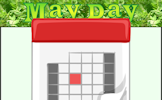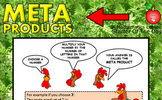# Area of trapezia and circles

### Term 3 starting in week 4 :: Estimated time: 2 weeks

• Calculate the area of triangles, rectangles and parallelograms (review)
• Calculate the area of a trapezium
• Calculate the perimeter and area of compound shapes (1)
• Investigate the area of a circle
• Calculate the area of a circle and parts of a circle without a calculator
• Calculate the area of a circle and parts of a circle with a calculator
• Calculate the perimeter and area of compound shapes (2)

This page should remember your ticks from one visit to the next for a period of time. It does this by using Local Storage so the information is saved only on the computer you are working on right now.

## Lesson Starters

Here are some suggestions for whole-class, projectable resources which can be used at the beginnings of each lesson in this block.

### 1st Lesson#### Icosahedron

How many triangles are there on the surface of a regular icosahedron.

### 2nd Lesson#### Tool Triangle

Place the numbers on the triangle so that the totals along each of the sides are equal.

### 3rd Lesson#### May Day

Add together the dates of all the Thursdays in May this year. Which day sum is largest?

### 4th Lesson#### Meta Products

Which numbers when multiplied by the number of letters in the word(s) of the number give square numbers?

### 5th Lesson#### News Common

Follow the compass directions given in the squares to find where the route starts?

### 6th Lesson#### One one

Continue the given number pattern with the help of a little lateral thinking.

Some of the Starters above are to reinforce concepts learnt, others are to introduce new ideas while others are on unrelated topics designed for retrieval practice or and opportunity to develop problem-solving skills.

White Rose ResourcesEnd of block assessments provide a quick progress check at the end of each block of learning to make sure students have understood the content covered. This Scheme of Learning was produced by White Rose Maths and is used here with permission granted on 30th June 2021.For All: